×#### Thank you for registering.

One of our academic counsellors will contact you within 1 working day.

Click to Chat

1800-1023-196

+91-120-4616500

CART 0

• 0

MY CART (5)

Use Coupon: CART20 and get 20% off on all online Study Material

ITEM
DETAILS
MRP
DISCOUNT
FINAL PRICE
Total Price: Rs.

There are no items in this cart.
Continue Shopping• Complete JEE Main/Advanced Course and Test Series
• OFFERED PRICE: Rs. 15,900
• View Details

```Chapter 8: Quadratic Equations Exercise – 8.12

Question: 1

A takes 10 days less than the time taken by B to finish a piece of work. If both A and B together can finish a work in 12 days, find the time taken by B to finish the piece of work.

Solution:

Let us consider B tales x days to complete the piece of work

B's 1 day work = 1/x

Now, A takes 10 days less than that of B to finish the same piece of work that is (x –10) daysSame work in 12 days

(A and B)'s 1 day's work = 1/12

According to the question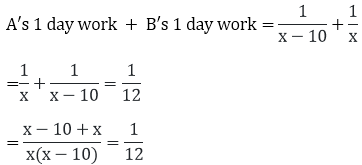= 12(2x - 10) = x(x - 10)

= 24x - 120 = x2 - 10x

= x2 - 10x - 24x + 120 = 0

= x2 - 34x + 120 = 0

= x2 - 30x - 4x + 120 = 0

= x(x - 30) - 4(x - 30) = 0

= (x - 30)(x - 4) = 0

Either x - 30 = 0 therefore x = 30

Or, x - 4 = 0 therefore x = 4

We observe that the value of x cannot be less than 10 so the value of x = 30

Time taken by B to finish the piece of work is 30 days

Question: 2

If two pipes function simultaneously, a reservoir will be filled in 12 hours. One pipe fills the reservoir 10 hours faster than the other. How many hours will the second pipe take to fill the reservoir?

Solution:

Let us assume that the faster pipe takes x hours to fill the reservoir

Portion of reservoir filled by faster pipe in one hour = 1/x

Now, slower pipe takes 10 hours more than that of faster pipe to fill the reservoir that is (x + 10) hours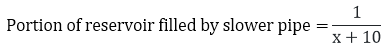Given that, if both the pipes function simultaneously, the same reservoir can be filled in 12 hours

Portion of the reservoir filled by both pipes in one hour = 1/12

Now,

Portion of reservoir filled by slower pipe in one hour + Portion of reservoir filled by faster pipe in oneAnd portion of reservoir filled by both pipes = 1/12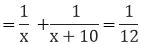= 12(2x + 10) = x(x + 10)

= x2 - 14x - 120 = 0

= x2 - 20x + 6x - 120 = 0

= x(x - 20) + 6(x - 20) = 0

= (x - 20)(x + 6) = 0

Either x - 20 = 0 therefore x = 20

Or, x + 6 = 0 therefore x = – 6

Since the value of time cannot be negative so the value of x is 20 hours

Time taken by the slower pipe to fill the reservoir = x + 10 = 30 hours

Question: 3

Two water taps together can fill a tank in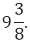The tap of larger diameter takes 10 hours less than the smaller one to fill the tank separately. Find the time in which each tap can be fill separately the tank.

Solution:

Let the time taken by the tap of smaller diameter to fill the tank be x hours

Portion of tank filled by smaller pipe in one hour = 1/x

Now, larger pipe diameter takes 10 hours less than the smaller diameter pipe in one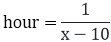Given that,

Two taps together can fill the tank in= 75/8.

Now, portion of the tank filled by both the taps together in one hour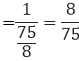We have,

Portion of tank filled by smaller pipe in one hour + Portion of tank filled by larger pipe in one hour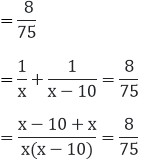= 75(2x - 10) = 8x(x - 10)

= 150x - 750 = 8x2 - 80x

= 8x2 - 230x + 750 = 0

= 4x2 - 115x + 375 = 0

Here a = 4, b = -115, c = 375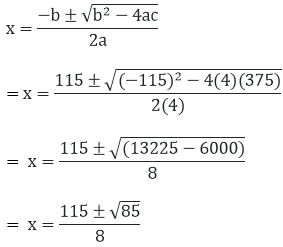The value of x can either be 8 or 3.75 hours.

The value of x is 8 hours

Question: 4

Two pipes running together can fill the tank in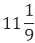minutes. If one pipe takes 5 minutes more than the other to fill the tank separately. Find the time in which each pipe would fill the tank separately.

Solution:

Let us take the time taken by the faster pipe to fill the tank as x minutes

Portion of tank filled by faster pipe in one minute = 1/x

Now,

Time taken by the slower pipe to fill the same tank is 5 minutes more than that of faster pipe = x + 5 minutes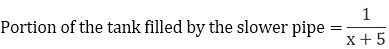Given that,Portion of tank filled by two pipes together in 1 minute = 9/100

Portion of tank filled by faster pipe in one minute + Portion of the tank filled by the slower pipe= 9x(x + 5) = 100(2x + 5)

= 9x2 + 45x = 200x + 500

= 9x2 - 155x - 500 = 0

= 9x2 - 180x + 25x - 500 = 0

= 9x(x - 20) + 25(x - 20) = 0

= (x - 20)(9x + 25) = 0

Either x - 20 therefore x = 20Since time cannot be negative

So the value of x = 20 minutes

The required time taken to fill the tank is 20 minutes

Time taken by the slower pipe is x + 5 = 20 + 5 = 25 minutes

Times taken by the slower and faster pipe are 25 minutes and 20 minutes respectively.
```### Course Features

• 728 Video Lectures
• Revision Notes
• Previous Year Papers
• Mind Map
• Study Planner
• NCERT Solutions
• Discussion Forum
• Test paper with Video Solution